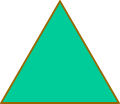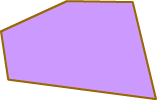# Regular and Irregular Polygons

Print
Rate 0 stars
Common Core
• Lesson size:
Message preview:
Someone you know has shared lesson with you:

To play this lesson, click on the link below:

https://www.turtlediary.com/lesson/regular-and-irregular-polygons.html

Hope you have a good experience with this site and recommend to your friends too.

Login to rate activities and track progress.
Login to rate activities and track progress.

A regular polygon is the one where all the sides are equal and all the angles are equal.An irregular polygon is one that is not regular.Let's go through some examples.

Example 1

Is this polygon regular or irregular?This triangle is a regular triangle. All three side lengths and angles are equal.

Example 2

Is this polygon regular or irregular?By counting the sides, we can see that this is a five sided figure. It is a pentagon. Since, the sides are not equal. Therefore, it is an irregular pentagon.

Summary
• A polygon is a closed figure made up of line segments.
• A regular polygon is the one where all the sides are equal and all the angles are equal.
• An irregular polygon is one that is not regular.

## More Shapes Lessons

Become premium member to get unlimited access.